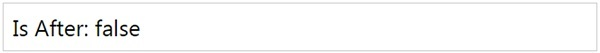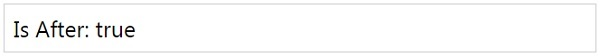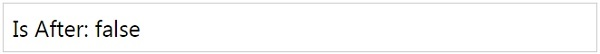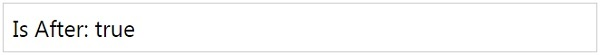# MomentJS - Is After

This method gives true or false if the given moment is after another moment.

## Syntax

```moment().isAfter(Moment|String|Number|Date|Array);
moment().isAfter(Moment|String|Number|Date|Array, String);
```

## Example

```var isafter = moment().isAfter();
```

## OutputIf no param to isAfter it considers it as the current date. It gives the output as false since the current date is not after the moment compared.

## Example

```var isafter = moment([2015, 10, 01]).isAfter([2014, 05, 10]);
```

## OutputIf the given date to isAfter is less, it gives true as the date is after the another moment.

## Example

```var isafter = moment([2015, 10, 01]).isAfter([2018, 05, 10]);
```

## OutputHere the date given to isAfter is greater than the date compared, so the output is false.

Consider the following examples to check isAfter() method with units.

## Example

```var isafter = moment([2015, 10, 01]).isAfter([2011, 05, 10], 'year');
```

## Outputmomentjs_date_queries.htm